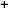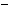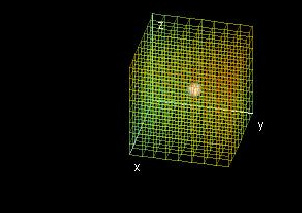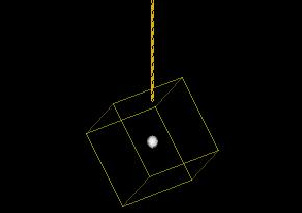Labware - MA35 Multivariable Calculus - Three Variable Calculus
 MA35 Labs 3 » Three Variable Calculus Contents3.4 Integration 3.4.5 Change of Variables 3.4.6 Center of Mass 3.4.7 Moment of Intertia Search

Center of Mass

Text

The center of mass of a three-dimensional region is a weighted average of the positions of the particles that the region comprises. The amount each particle counts in this weighted average is proportional to its mass.If we wanted to find the average position of a series of particles, we could find each coordinate of the point representing average position by adding up the values of that coordinate from each point and dividing by the number of points. For example, to find the x coordinate X in the average position of n particles, we would calculate

nxn)/n

Where xn is the x coordinate of the position of the nth particle.

Suppose instead we wanted to find the center of mass of a region as described in the first paragraph. Our expression for the x coordinate of the center of mass, given the mass mn of each particle is:

nxnmn)/(Σnmn)

(Note that the denominator is equal to the total mass of the set of particles.)

Given a density function ρ(x, y, z), we can express this summation as

nxn*ρ(xn, yn, zn)*An)/(Σnρ(xn, yn, zn)*Vn)

Where Vn is the volume of the nth particle.

For a region with domain D composed of a very large number of particles, we can change this summation to a double integral, replacing Vn with dV:

X = (∫∫∫Dx*ρ(x, y, z)dV)/(∫∫∫Dρ(x, y, z)dV).

Similarly, to find the y coordinate of center of mass, use:

Y = (∫∫∫Dy*ρ(x, y, z)dV)/(∫∫∫Dρ(x, y, z)dV)

and to find the z coordinate, use

Z = (∫∫∫Dz*ρ(x, y, z)dV)/(∫∫∫Dρ(x, y, z)dV).

This gives us the center of mass (X, Y, Z) of D.Demos

 Center of Mass: Rectangular PrismThis demo graphs a density function ρ(x, y, z) for a rectangular region and then finds the center of mass of that region. Changing "rhoMin" (estimated minimum value of ρ(x, y, z) and "rhoMax" estimated maximum value of ρ(x, y, z)) is optional. Doing so will improve the coloring of the graph (cyan at "rhoMin", red at "rhoMax").Center of Mass: Region Between Function GraphsThis is a variation of the previous demo. This time, you can use any region such that zMin (x, y) ≤ z ≤ zMax(x, y), yMin(x) ≤ y ≤ yMax (x), and x is between two constants.3D Objects on StringsIf you hang an object from a string, its center of mass will be under the string at rest position. In this demo, you can see this principle in action. As before, choose a density function ρ(x, y, z), two constants for the bounds of x, two functions for the bounds of y in terms of x, and two functions for the bounds of z in terms of x and y. You can then change x0 to see what happens when you attach the string at different points on the upper boundary of the object.Exercises

• 1. Does the center of mass change if the density function is multipied by some constant k? Why or why not?
• 2. Find a region and some density function ρ(x, y, z) such that the center of mass lies outside the region. In general, what geometric property must a region have for its center of mass to be able to lie outside of the region?